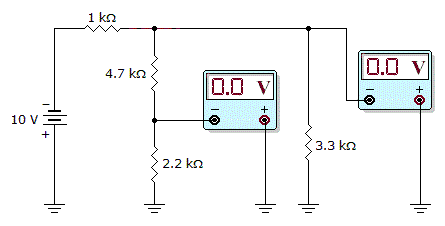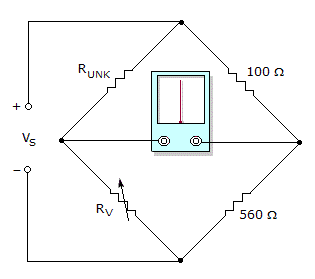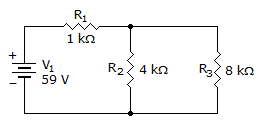# Electronics - Series-Parallel Circuits

### Exercise :: Series-Parallel Circuits - General Questions

31.Which circuit fault do the meter readings in the given figure indicate?

 A. The 1 kresistor is shorted. B. The 4.7 kresistor is shorted. C. The 2.2 kresistor is shorted. D. The 3.3 kresistor is shorted.

Explanation:

No answer description available for this question. Let us discuss.

32.

In a series–parallel circuit consisting of R1 and R2 in series and connected in parallel with R3, if R1 opens, then R2's voltage will:

 A. increase B. decrease C. remain the same D. cannot tell

Explanation:

No answer description available for this question. Let us discuss.

33.In the given circuit, what is RUNK equal to if RV must be adjusted to 1,232in order to balance the bridge?

 A. 220B. 6,899C. 1,232D. More information is needed in order to find RUNK.

Explanation:

No answer description available for this question. Let us discuss.

34.

With 6 V applied, what is the voltage across R2 of a parallel combination of R2 (1 k), R3 (2 k), and R4 (1 k) in series with R1, whose value is 2 k?

 A. 1 V B. 3 V C. 5 V D. 6 V

Explanation:

No answer description available for this question. Let us discuss.

35.

What is the power dissipated by R1, R2, and R3?A. P1 = 0.13 W, P2 = 0.26 W, P3 = 0.12 W B. P1 = 0.26 W, P2 = 0.52 W, P3 = 0.23 W C. P1 = 0.52 W, P2 = 0.92 W, P3 = 0.46 W D. P1 = 1.04 W, P2 = 1.84 W, P3 = 0.92 W